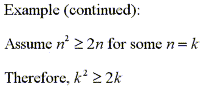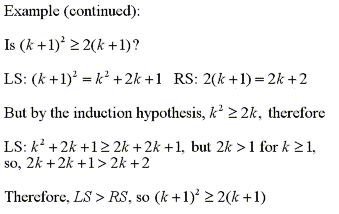TUTORIALS
TUTORIALS HOME

GENERAL MATH
NUMBER SETS
ABSOLUTE VALUE & INEQUALITIES
SETS & INTERVALS
FRACTIONS
POLYNOMIALS
LINEAR EQUATIONS
GEOMETRY
FINITE SERIES
TRIGONOMETRY
EXPONENTS
LOGARITHMS
INDUCTION

CALCULUS
LIMITS
DERIVATIVES
RELATED RATES & OPTIMIZATION
CURVE SKETCHING
INTEGRALS
AREA & VOLUME
INVERSE FUNCTIONS

MAIN
HOME
TESTS
TUTORIALS
PROBLEMS
COMMON MISTAKES
STUDY TIPS
GLOSSARY
CALCULUS APPLICATIONS
MATH HUMOUR

# Mathematical Induction

Mathematical induction is a powerful, yet straight-forward method of proving statements whose "domain" is a subset of the set of integers. Usually, a statement that is proven by induction is based on the set of natural numbers. This statement can often be thought of as a function of a number n, where n = 1,2,3...

Proof by induction involves three main steps: proving the base of induction, forming the induction hypothesis, and finally proving that the induction hypothesis holds true for all numbers in the domain.

Proving the base of induction involves showing that the claim holds true for some base value (usually 0, 1, or 2). There are sometimes many ways to do this, and it can require some ingenuity. We will outline this with a simple example.This is the base step. We simply prove that the statement holds true for at least one value of n. In the induction hypothesis step, we say that since the statement holds true for at least one value, we can assume that it will hold true for some arbitrary, fixed value of n, usually k. We can make this assumption since we know that it will at least be true for our base value (2 in our example)This is the simplest, yet most powerful part of proof by induction. By forming the induction hypothesis, we can now use it to finish the proof.

In the final step, we prove that the induction hypothesis holds true for all values of n. To do this, we use the fact that the statement is true for n = k, and then check to see if it holds true for n = k + 1.Using this fact, we can now state that n²≥2n for all n = 2, 3... At first it may seem too simple, but if you examine this proof, it is easy to see the logic behind it. We know that the statement is true for n = 2. So we can now assume that it is true up to some fixed number k, which is at least 2. By proving that it is true for k + 1, we now know that it is true for at least n = 3. So now k = 3, but since the statement is true for k + 1, n is at least 4. In this manner, we can repeat this pattern indefinitely. Therefore, the statement is proven true for all n.

While different statements can require different techniques to prove that the statement is true (in the base step and k + 1 step), the reasoning behind a proof by induction is always the same. You must always follow the three steps:

1) Prove the statement true for some small base value
(usually 0, 1, or 2)
2) Form the induction hypothesis by assuming the statement is true
up to some fixed value n = k
3) Prove the induction hypothesis holds true for n = k + 1

There is one very important thing to remember about using proof by induction. This technique can only be used to prove statements that have real valued inputs. If you think of the statement as a relation of functions (for example, prove f(x) ≤ g(x) for all x), the domain of these functions must be a subset of the integers. It cannot be used to prove statements true for non-integer values. For example, the proof that we did in the above example does not prove that (2.5)² ≥ 2(2.5). It only proves the statement true for integer values of k greater than 2 (k = 2, 3, ...) However, for many proofs involving statements based on subsets of the integers (usually natural numbers), proof by induction is the easiest method to use.

# Examples

1 | Prove the formula for the sum of the first n natural numbers

| Top of Page |

COURSE HOMEPAGES
MATH 1036
MATH 1037

FACULTY HOMEPAGES
Alex Karassev
Ted Chase